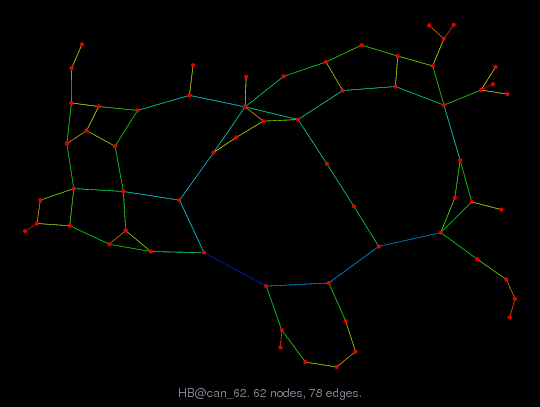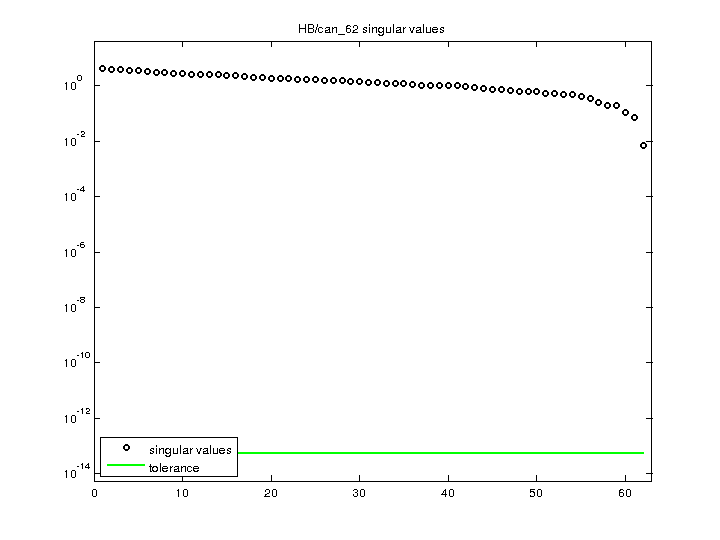Matrix: HB/can_62

Description: SYMMETRIC PATTERN FROM CANNES,LUCIEN MARRO,JUNE 1981.(undirected graph drawing)• Matrix group: HB
• download as a MATLAB mat-file, file size: 872 bytes. Use UFget(103) or UFget('HB/can_62') in MATLAB.

 Matrix properties number of rows 62 number of columns 62 nonzeros 218 structural full rank? yes structural rank 62 # of blocks from dmperm 1 # strongly connected comp. 1 explicit zero entries 0 nonzero pattern symmetry symmetric numeric value symmetry symmetric type binary structure symmetric Cholesky candidate? yes positive definite? no

 author L. Marro editor I. Duff, R. Grimes, J. Lewis date 1981 kind structural problem 2D/3D problem? yes

 Ordering statistics: result nnz(chol(P*(A+A'+s*I)*P')) with AMD 184 Cholesky flop count 5.9e+02 nnz(L+U), no partial pivoting, with AMD 306 nnz(V) for QR, upper bound nnz(L) for LU, with COLAMD 203 nnz(R) for QR, upper bound nnz(U) for LU, with COLAMD 361

 SVD-based statistics: norm(A) 4.15908 min(svd(A)) 0.00688791 cond(A) 603.824 rank(A) 62 sprank(A)-rank(A) 0 null space dimension 0 full numerical rank? yes

 singular values (MAT file): click here SVD method used: s = svd (full (A)) ; status: ok